# Fourier series calculatorExpansion of some function f(x) in trigonometric Fourier series on interval [-k, k] has the form: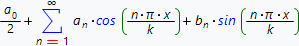where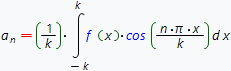для (n = 0, 1, 2, 3,...)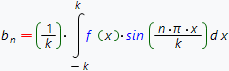для (n = 1, 2, 3,...)

As an example, find Fourier series expansion of the function f(x)=x on interval [-1, 1]. In this case the coefficients an и bn are determined by the formulas: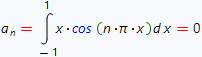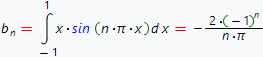Therefore, the expansion of function f(x)=x in Fourier series on interval [-1, 1] has the form: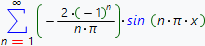We can see two plots on the figure below f(x)=x (yellow color) and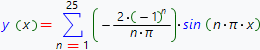, (blue color) for which we use order of expansion equal to 25.It should be noted, that in example above, the coefficients an are zero not by chance. The fact is that function f(x)=x is odd on interval [-1, 1]. In contrast, the function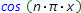- is even. The product of an even function by the odd one is the odd function, so according to the properties , integral of the odd function on symmetric interval is zero.

In case of the even function, for example x2 , coefficients bn were zero, because the integrand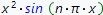- is odd function.

Based on the above reasoning, we can draw the following conclusions:

• Fourier series expansion of an odd function on symmetric interval contains only sine terms.
• Fourier series expansion of an even function on symmetric interval contains only cosine terms.
• If we need to obtain Fourier series expansion of some function on interval [0, b] , then we have two possibilities. We can continue this function on interval [-b, 0] in an odd way and then only sines terms will present in expansion. Or we can continue this function on interval given above in an even way and then only cosine terms will present in expansion.

It should be noted, that by using the formulas given above and corresponding variable substitution, it is possible to obtain the formulas for Fourier series expansion coefficients of some function at an arbitrary interval. [p, q]: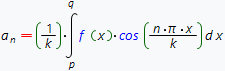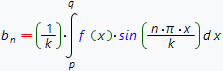here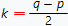.

Our online calculator, build on Wolfram Alpha system finds Fourier series expansion of some function on interval [-π π]. In principle, this does not impose significant restrictions because using the corresponding variable substitution we can obtain an expansion at an arbitrary interval [p, q].

Fourier series calculator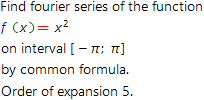Function to find Fourier series expansion